Solving differential equations with step functions

Solving differential equations with step functions

Heaviside step functions

On this lesson we will be looking one of the main uses of a step function, and that is to use it as a tool to solve differential equations. And so, we will take from different lessons we have seen already along the way, mainly from the lesson about step functions, but also looking into the Laplace equation for special cases, such as the Laplace of y'', etc. But first, let us begin with a little review on the basic concepts:

The Heaviside function, also known as the unit step function, is a function that is defined to have a constant value of zero up to a certain point on t (the horizontal axis) at which it jumps to a value of 1. This particular value at which the function jumps (or switches from zero to one) is usually taken as the origin in the typical coordinate system representation, but it can be any value c in the horizontal axis. Below you can see the usual representations you will find in problems and so, on how to graph step functions:

The Heaviside step function is defined as: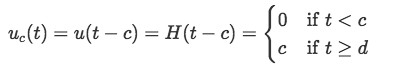Equation 1: Definition of the Heaviside function or unit step function.

Where c represents the point at t in which the function goes from a 0 value to a value of 1. So, if we want to write down the mathematical expression of the heaviside function depicted in figure 3, we would write it as: u3(t). Notice how we only wrote the math expression using the first type of notation found in equation 1, this is because that notation happens to be the most commonly used and is also the one we will continue to use throughout our lesson. Is still important though, that you know about the other notations and keep them in mind just in case you find them throughout your studies.

From the unit step function equation (equation 1), we can understand why the Heaviside function is also called the "unit step" function. As it can be observed, a Heaviside function can only have values of 0 and 1, in other words, the function is always equal to zero before arriving to a certain value t=c at which it "turns on" and jumps directly into having a value of 1, and so, it jumps in a step size of one unit.

Notice how we have used the phrase "turning on" to describe the process of the unit step function to jump from a zero value to a unit value, this is a very common way to refer to Heaviside step functions' behavior and interestingly enough, it is all due to their real life usage and comes from the reason why they were invented. Historically, physicist, self-taught engineer and mathematician Oliver Heaviside, invented Heaviside step functions in order to describe the behaviour of a current signal when you click the switch on of an electric circuit, thus, allowing you to calculate the magnitude of the current from zero when the circuit is off, to a certain value when is tuned on.

Although the Heaviside function itself can only have the values of 0 or 1 as mentioned before, this does not mean we cannot obtain a graphic representation of a higher jump using Heaviside step functions. It is actually a very simple task to obtain a higher value since you just need to multiply it for any constant value that you want to have as the size jump, in other words, if you have a step function written as 3u5(t) in here you have a step function which has a value of zero until it gets to a value of t=5, and which point, the function has a final value of 3. This can be seen in the step graph below:

One of the greatest properties of Heaviside step functions is that they allow us to model certain scenarios and mathematically be able to solve for important information on these scenarios. We have talked about such Heaviside function examples before in our past lesson. This lesson is intended to bring some more in depth knowledge on how to solve such unit step function examples where we tend to require the use of the Laplace transform in order to solve differential equations.

This actually becomes the most important point of the lesson for today, and after you have had an introduction and worked on step function examples, now is time to solve differential equations with them. The process is lengthy, thus, we will focus on one example only; before you get to that point, we want to make sure you have already studied the lesson on solving differential equations with the Laplace transform, since not only the methodology will be similar, but also we will borrow a set of formulas from that lesson, which you should be already acquainted with.

We will talk a bit more about this on the last section for this lesson, meanwhile for a review on the definition of the unit step function and a list of a few of its identities, we recommend the next Heaviside step function article.

Laplace transform of Heaviside function

You have already had an introduction to the Laplace transform in recent past lessons, still, at this time we do recommend you to give the topic a review if you think it appropriate or necessary. The lesson on calculating Laplace transforms is of special use for you to be prepared for this section.

Let us continue with the Heaviside step function and how we will use it along the Laplace transform. The Laplace transform helps us find the value of y(t) for a function that is represented using the unit step function (mostly shifted functions).

These shifted functions will be equal to zero until at certain point c in which they will "turn on", they can be represented as:

The shifted function then is defined as:

Now let's see what happens when we take the Laplace transform of a shifted function! First, remember that the mathematical definition of the Laplace transform is: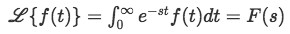Equation 3: Laplace transform for a function f(t) where t ? 0.

Therefore the Laplace transform for the shifted function is: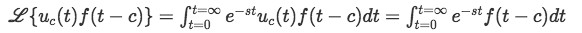Equation 4: Laplace transform of shifted function (part 1).

Notice how the Laplace transform gets simplified from an improper integral to a regular integral where you have dropped the unit step function. The reason for this is that although the range of the whole Laplace transform integral is from 0 to infinity, before c (whatever value c has) the unit step function is equal to zero, and therefore the whole integral goes to zero. After c, the unit step function has a value of 1, and thus, we can just take it as a constant value of 1 multiplying the rest of the integral, which range is not from c to infinity.

Continuing with the simplification of the Laplace transform of the shifted function, we set x = t - c, which means that t = x+c and so the transformation looks as follows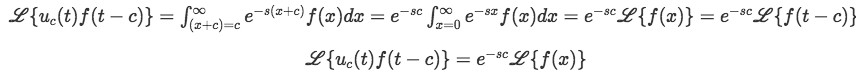Equation 5: Laplace transform of shifted function (part 2).

All of these computations can be found in the past lesson, but since they are of relevance for the example we will working up today, let us continue with another important equation to know: the unit step function Laplace transform. Notice, not the transform for a shifted function, but the Laplace transform of the unit step function (Heaviside function) itself and alone.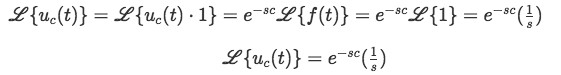Equation 6: Laplace transform of the unit step function (Heaviside step function)

Notice, equation 5 was useful while obtaining equation 6 because taking the Laplace transformation of the Heaviside function by itself can be taken as having a shifted function in which the f(t-c) part equals to 1, and so you end up with the Laplace transform of a unit step function times 1, which results in the simple and very useful formula found in equation 6.

Now the interesting part of this lesson comes from us not necessarily using the Laplace transform in our computations, actually we will be using the inverse Laplace transform (for which we will explain its formula while working through the problem so you can follow the steps along and the reasoning behind its usage).

Laplace transform of step function (example)

For this part, we will be needing a few extra bits of information from past lessons. First, we go to the lesson on solving differential equations with the Laplace transform and take the two important and useful formulas: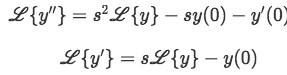Equation 7: Laplace transforms of the derivatives of y

And also, an item which has been useful for so many lessons already and which we hope you have gotten used to working with: the table of Laplace transforms.

Example

Solve the following differential equation using the initial conditions y(0)=3, y'(0)=2.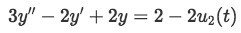Equation 8: Second order constant coefficient, nonhomogeneous

We start by taking the Laplace transform of each of the terms in equation 8: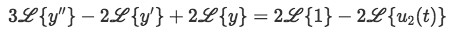Equation 9: Laplace transform of the differential equation

The easiest part to solve is the right hand side, so let us start by solving that: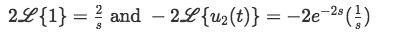Equation 10: Final result for the right hand side

Now we work out the Laplace transforms on the left hand side of equation 9 using equations 7: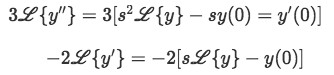Equation 11: Laplace transforms for the derivatives of y

And so we rewrite equation 9 completely: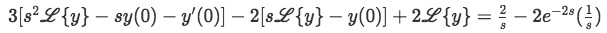Equation 12: Rewriting the Laplace transform equation 9

Applying the initial conditions: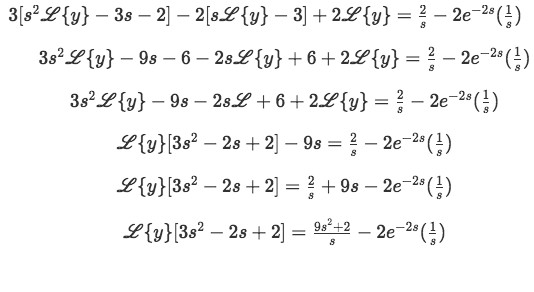Equation 13: Simplifying the equation using the initial conditions

And so, we solve for the Laplace transform of y by passing the factor in front of it to the right hand side of the equation, we do this of course by dividing both sides by the whole factor to obtain: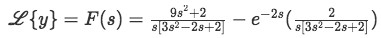Equation 14: Laplace transform of y

In order to obtain y, we will have to apply the inverse Laplace transform to the whole equation 14. Since this process will get lengthy, we will do it by parts, starting by solving the inverse Laplace transform for the first term on the right. Due to the nature of the mathematical expressions we are solving, we will use the method of partial fractions to simplify the equation until is easier to resolve using the method of comparison with the table.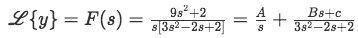Equation 15: Simplifying by partial fractions (part 1)

Therefore, we solve for the coefficients A, B and C: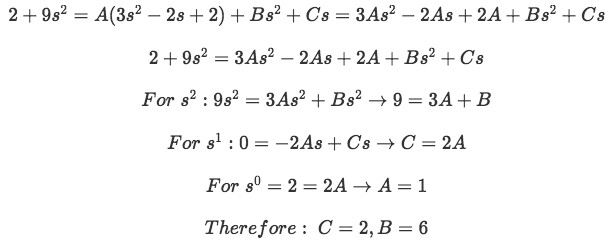Equation 16: Simplifying by partial fractions (part 2)

And so, rewriting equation 15 with what we have found we obtain: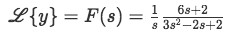Equation 17: Laplace transform of y

To find the value of y now, we just have to find the inverse Laplace transform and we do this by method of comparison with table 1:

We have finished solving the first term on the right hand side of equation 14, now let us finish this problem by solving the inverse Laplace transform for the second term in the right hand side: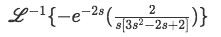Equation 19: Inverse Laplace transform of second term from equation 14

Let us simplify the function inside the inverse transformation using partial fractions decomposition: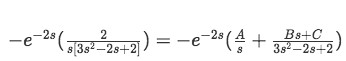Equation 20: Simplifying by partial fractions (part 1)

Therefore, the equations to solve the coefficients A, B and C go as follows: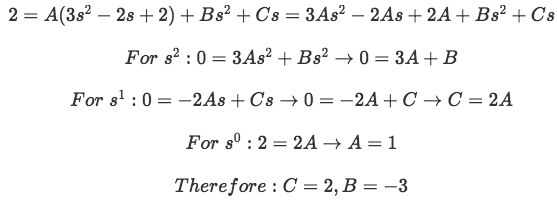Equation 21: Simplifying by partial fractions (part 2)

We rewrite equation 20 and simplify as possible: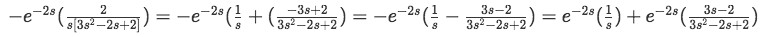Equation 22: Simplifying thanks to partial fractions (part 3)

Now we can obtain the inverse Laplace transform of the second term in equation 14 (which has been simplified to the expression in equation 22):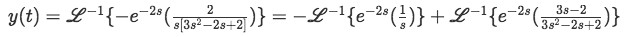Equation 23: Inverse Laplace transform for second term in equation 14

In order to solve this last equation, we take the hint from the terms we can find on it. In other words, just by looking at the exponential factor we can directly know that the function which would produce such a result from its Laplace transform contained a unit step function, and thus, we go back to our useful equation to find out that we can use equation 5 to obtain the result for this inverse transformation: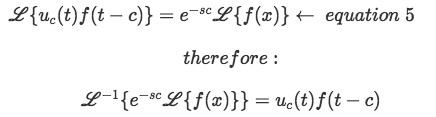Equation 24: Inverse Laplace transform which produces a unit step function

Taking equation 24 as our main tool, we notice the first term on the right hand side of equation 23 is very simple to solve, so we start by solving the inverse transform on it. Then we look for an equivalent expression for the second term in the right hand side so we can simplify it as much as possible and solve it by comparison with table 1.

This process is similar to what we did on equation 18, we advise you to pay attention to our lesson video so you can see how these "simplified" expressions are obtained. At this point you should have extended practice in using table 1, and so, you probably know that this process of obtaining a "simplified" expression in order to use the method of comparison with table 1 requires mostly intuition and skill obtained by having done these kinds of problems many times. Therefore, we have summarized the computations in here, but if any doubts arise about how to solve this problem, rely on the video lesson to explain it extensively.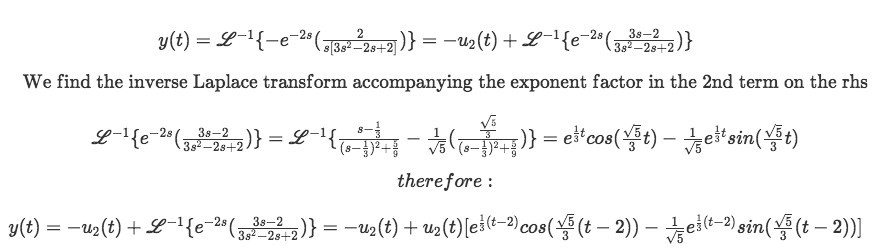Equation 25: Solving the inverse Laplace transform for expression found in equation 22

And so, we can finally write down the complete solution to equation 4. This full solution to the problem is obtained by adding together equations 18 and 25 as follows: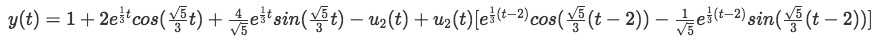Equation 26: Final solution to the differential equation

And so, we have come to the end of our lesson. Before passing to the next lesson in our course for differential equations, we recommend you to check out the next Heaviside function, Dirac Delta function little article, so you can start looking at the relationship, similarities and differences between these two topics, since your next lesson will be the Dirac delta function itself.

Solving differential equations with step functions

Lessons

We can use Laplace Transforms to solve second order differential equations with step functions

The following formulas may be needed:

$L${$y$''} = $s^{2}$$L${$y$} - s $y$(0) - $y$'(0)

$L${$y$'} = s $L${$y$} - $y$(0)

$L${$u_{c}(t)$$f(t - c)$} = $e^{-sc}$$L${$f(t)$}

• 1.
Solving Differential Equations with Step Functions Using the Laplace Transform

Solve the following differential equation:

$3y'' - 2y' + 2y = 2 - 2u_{2}(t)$

Using initial conditions $y$(0) = 3, $y$'(0) = 2